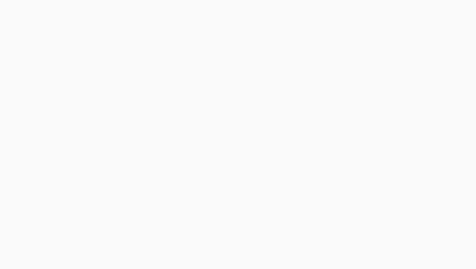# Which is the best way to estimate measure of photographed things?

1. find the coin (`green` bounding box rectangle)

either manually or by some search for specific color,pattern,hough transform,segmentation… This will limit the area to search for next steps

2. find the boundary (distinct `red` edge in color intensity)

so create a list of points that are the coin boundary (be careful with shadows) just scan for high enough intensity bumps.

3. compute the circle center

just average of all border points…

4. test all boundary points for `min/max` distance to center

if the tilt is small then you will have many points with min and max radius so take the middle from them. If the `|max-min|` is very small then you got no tilt. Linebetween min/max distance point and center gives you `black` basis vectors.

5. use `black` basis vectors to measure

So select 2 points (`red` line d) to measure and cast `green` rays from them parallel to basis vectors. Their intersection will create `2` lines `a,b`. from that it is easy:

• `d = sqrt((a*a)+(b*b))`

where `a,b` is the size of the lines in units. you can obtain it like:

• `a_size_unit = a_size_pixel * coin_r_unit / rmax_pixel`
• `b_size_unit = b_size_pixel * coin_r_unit / rmin_pixel`[note]

This image was selected to emphasize the skew but you should use images of planes almost paralel to chip surface to avoid perspective distortion. This image is not a good example the cube is more distant to camera then coin …

To account for this see selection criteria for different projections

Categories ios